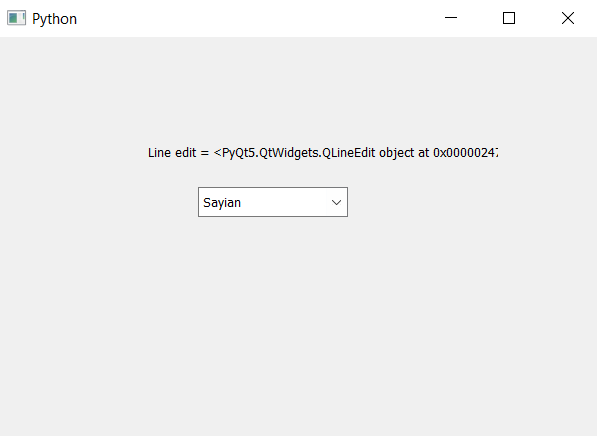Related Articles
PyQt5 – How to get line edit of ComboBox
• Last Updated : 22 Apr, 2020

In this article we will see how we can get the line edit of combo box, line edit is basically a default object of combo box where we insert the text, it is used by the combo box to show the selected option and for getting the input, we can get the line edit object of the combo box with the help of `setLineEdit` method

In order to get the delegate object of the combo box we will use `lineEdit` method

Syntax : combo_box.lineEdit()

Argument : It takes no argument

Return It returns QLineEdit object

Below is the implementation –

 `# importing libraries ` `from` `PyQt5.QtWidgets ``import` `*`  `from` `PyQt5 ``import` `QtCore, QtGui ` `from` `PyQt5.QtGui ``import` `*`  `from` `PyQt5.QtCore ``import` `*`  `import` `sys ` ` `  ` `  `class` `Window(QMainWindow): ` ` `  `    ``def` `__init__(``self``): ` `        ``super``().__init__() ` ` `  `        ``# setting title ` `        ``self``.setWindowTitle(``"Python "``) ` ` `  `        ``# setting geometry ` `        ``self``.setGeometry(``100``, ``100``, ``600``, ``400``) ` ` `  `        ``# calling method ` `        ``self``.UiComponents() ` ` `  `        ``# showing all the widgets ` `        ``self``.show() ` ` `  `    ``# method for widgets ` `    ``def` `UiComponents(``self``): ` ` `  `        ``# creating a combo box widget ` `        ``self``.combo_box ``=` `QComboBox(``self``) ` ` `  `        ``# setting geometry of combo box ` `        ``self``.combo_box.setGeometry(``200``, ``150``, ``150``, ``30``) ` ` `  `        ``# geek list ` `        ``geek_list ``=` `[``"Sayian"``, ``"Super Saiyan"``, ``"Super Sayian 2"``, ``"Super Sayian B"``] ` ` `  `        ``# adding list of items to combo box ` `        ``self``.combo_box.addItems(geek_list) ` ` `  `        ``# creating a line edit ` `        ``edit ``=` `QLineEdit(``self``) ` ` `  `        ``# setting line edit ` `        ``self``.combo_box.setLineEdit(edit) ` ` `  `        ``# getting line edit ` `        ``line ``=` `self``.combo_box.lineEdit() ` ` `  `        ``# creating label to show delegate ` `        ``label ``=` `QLabel(``"Line edit = "` `+` `str``(line), ``self``) ` ` `  `        ``# setting geometry of the label ` `        ``label.setGeometry(``150``, ``100``, ``350``, ``30``) ` ` `  ` `  `# create pyqt5 app ` `App ``=` `QApplication(sys.argv) ` ` `  `# create the instance of our Window ` `window ``=` `Window() ` ` `  `# start the app ` `sys.exit(App.``exec``()) `

Output :Attention geek! Strengthen your foundations with the Python Programming Foundation Course and learn the basics.

To begin with, your interview preparations Enhance your Data Structures concepts with the Python DS Course.

My Personal Notes arrow_drop_up
Recommended Articles
Page :